# A new way to experience math

Exclusive content and tools teachers need to create powerful learning experiences.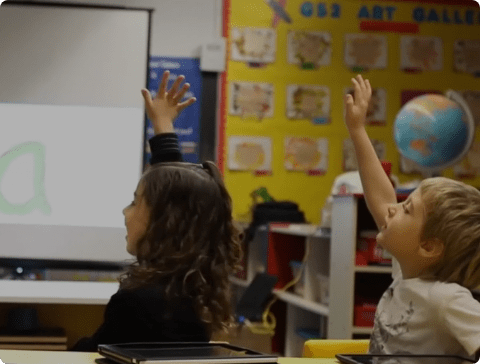## Maximize outcomes for every student

Nearpod Math, our K-8 supplemental curriculum, helps teachers enhance instruction with intentionally organized content and tools to create engaging math learning experiences for every student.

Finally, a supplemental program focused on the instructional experience.

• Search, download, and customize K-8 standards-based math resources built using the Nearpod experience.

•• Encourage exploration, inquiry, and conversations about math through pedagogical strategies and exclusive features like virtual math manipulatives.

•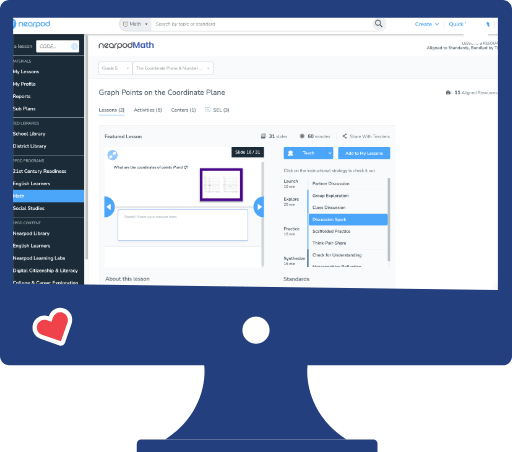• Help students see themselves as mathematicians with relevant, engaging, and challenging experiences that apply math concepts to real-world scenarios.

•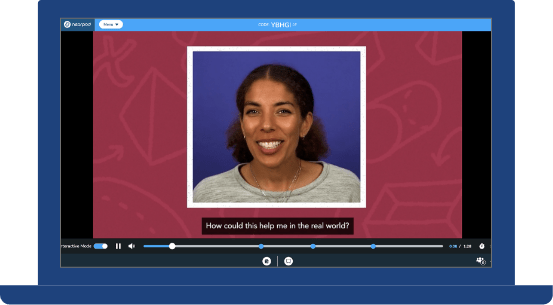• Meet the varied needs in each classroom by incorporating student voice, creating safe spaces to participate, and getting insights into student understanding.

•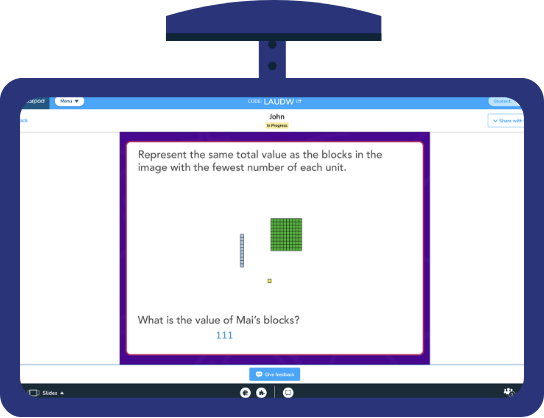••••## Enough content to support any instructional decision you make to differentiate learning, everyday.

• ### Math Manipulatives

• Engage the whole class and cover key math concepts with 40-60 minute problem-based math lessons.

• Quickly reinforce and practice key concepts covered in your whole class instruction.

• K-5 lessons offering gamified experiences and designed for collaborative group work.

• Help students visualize abstract math concepts with pre-built and customizable Color Tiles, Fraction Tiles, Algebra Tiles, and Base Ten Blocks.

## Enough content to support any instructional decision you make to differentiate learning, everyday.

• ### Problem-based lessons

Engage the whole class and cover key math concepts with 40-60 minute problem-based math lessons.

• ### Practice lessons and activities

Quickly reinforce and practice key concepts covered in your whole class instruction.

• ### Centers

K-5 lessons offering gamified experiences and designed for collaborative group work.

• ### Math Manipulatives

Help students visualize abstract math concepts with pre-built and customizable Color Tiles, Fraction Tiles, Algebra Tiles, and Base Ten Blocks.

1.2 million teachers around the world love teaching with Nearpod, collecting 1.5 billion real-time student insights last year.

## Preview lessons, videos, and activities exclusive to Nearpod Math.

•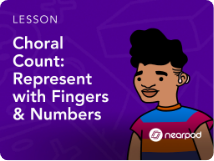#### In this collection of Kindergarten resources, students build toward mastery of:

• Verbally counting by ones from 1 to 100
• Verbally counting by tens from 10 to 100
• Counting to 10 from given numbers or identifying missing numbers in the count sequence to 10
• Counting to 100 from given numbers, or identifying missing numbers in the count sequence to 100
• Organizing numerals or groups of images in order from least to greatest or greatest to least
•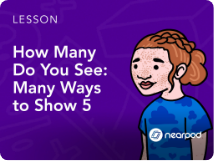#### In this collection of Kindergarten resources, students build toward mastery of:

• Recognizing and representing numbers up to 5 in various forms
• Recognizing and representing numbers up to 10 in various forms
• Recognizing and representing numbers up to 20 in various forms
•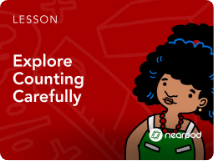#### In this collection of Kindergarten resources, students build toward mastery of:

• Physically moving a group of up to 10 items to count out, and representing the quantity with a numeral
• Representing the quantity of a static group of images by assigning the group a number
• Counting and matching given groups of images with the same quantity in different arrangements
• Reproducing the same number or set of objects in different arrangements
• Quickly recognizing the total number of objects or shapes in a set without counting and assigning a number name to the total
• Making or identifying groups of one more and one less
• Using ordinals from first through fifth
•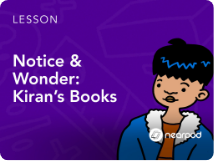#### In this collection of first grade resources, students build toward mastery of solving:

• Add to and take from problems within 10 that have the start, change, and result unknown using objects, drawings, and equations
• Put together and take apart problems within 10 that have an addend or total unknown using objects, drawings, and equations
• Compare problems within 10 that have the difference, bigger amount, and smaller amount unknown using objects, drawings, and equations
•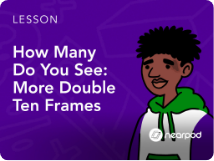#### In this collection of first grade resources, students build toward mastery of:

• Fluently adding and subtracting within 10
• Adding within 20 by making 10
• Subtracting within 20 by decomposing a number leading to a 10
• Adding and subtracting within 20 by using the relationship between addition and subtraction and showing counting on and counting back on number lines
• Adding and subtracting within 20 using base ten diagrams and ten frames to model regrouping
• Adding and subtracting within 20 using various strategies, including using manipulatives to create models
•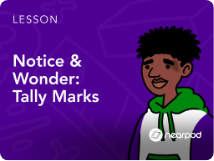#### In this collection of first grade resources, students build toward mastery of:

• Measuring, ordering, and comparing objects or distance using non-standard units
• Telling and writing time to the nearest hour and half hour
• Creating tally charts and tables, and answering questions about the data points
•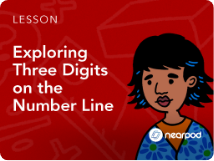#### In this collection of second grade resources, students build toward mastery of:

• Skip counting by 5s, 10s, and 100s within 1,000
• Composing and decomposing three-digit numbers within 1,000
• Writing and identifying the expanded form and word form of three-digit numbers
• Comparing and ordering three-digit numbers
•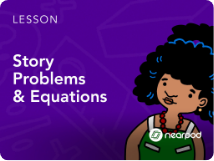#### In this collection of second grade resources, students build toward mastery of:

• Solving one-step real-world add to, take from, put together, and take apart problems within 100
• Solving one- and two-step real-world compare problems within 100
• Writing and identifying equations for one-step real-world problems within 100, using a symbol to represent the unknown quantity
• Using equations and diagrams to solve up to two-step real-world problems within 100
•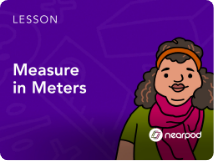#### In this collection of second grade resources, students build toward mastery of:

• Estimating lengths using units of inches, feet, centimeters, and meters
• Measuring the length of objects by selecting and using appropriate tools
• Measuring objects to determine the difference in length
• Understanding the inverse relationship between the size of the unit and the number of units needed to equal the length of an object
• Solving one- and two-step measurement problems within 100 involving lengths in the same unit
• Representing whole numbers as distances on number line diagrams
• Creating and interpreting line plots to display length measurement data
• Representing and interpreting data sets with up to four categories using picture graphs and bar graphs with single-unit scale
•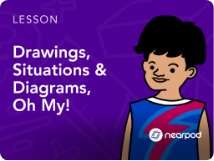#### In this collection of third grade resources, students build toward mastery of understanding:

• Multiplication as equal groups by interpreting and creating models
• Multiplication as equal groups by creating or interpreting arrays
• Multiplication by creating or interpreting models using expressions and equations
• Multiplication expressions and equations by creating or interpreting arrays
•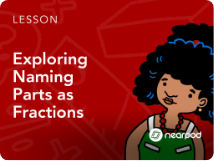#### In this collection of third grade resources, students build toward mastery of:

• Partitioning shapes into parts with equal areas and expressing the area of each part as a unit fraction
• Understanding a fraction 1/b as the quantity formed by 1 part when a whole is partitioned into b equal parts
• Understanding a fraction a/b as the quantity formed by a parts of size 1/b
• Identifying and representing unit fractions on number lines
• Identifying or representing non-unit fractions on number lines
•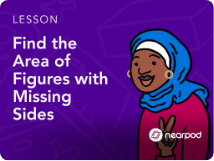#### In this collection of third grade resources, students build toward mastery of:

• Recognizing area as an attribute of plane figures
• Modeling the area of figures by covering figures in square units
• Determining the area of tiled figures and representing the area in square units
• Recognizing relationships between standard units of measurement
• Understanding the area of rectangles
• Multiplying side lengths to find areas of rectangles in the context of solving real-world and mathematical problems
• Using area models to represent the distributive property in mathematical reasoning
• Finding the area of rectilinear figures by decomposing them into non-overlapping rectangles and adding the areas of the non-overlapping part
• Finding unknown side lengths and areas of rectilinear figures
•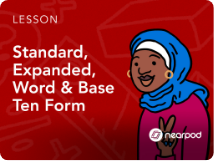#### In this collection of fourth grade resources, students build toward mastery of:

• Reading, writing, and representing multi-digit whole numbers through one billion in standard form, expanded form, and word form
• Recognizing that in a multi-digit whole number, a digit in one place represents 10 times what it represents in the place to its right and one-tenth what it represents in the place to its left
• Comparing and ordering multi-digit whole numbers based on meanings of the digits in each place, using comparison symbols to record the results of comparisons
• Rounding multi-digit whole numbers to any place
•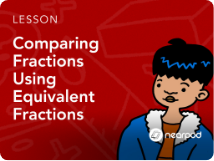#### In this collection of fourth grade resources, students build toward mastery of:

• Identifying and generating equivalent fractions using manipulatives, visual models, number lines, and equations
• Comparing fractions by creating equivalent fractions using benchmark fractions, manipulatives, visual models, number lines, and equations
• Comparing and ordering more than two fractions by creating equivalent fractions using benchmark fractions, manipulatives, visual models, number lines, and equations
• Decomposing non-unit fractions, improper fractions, and mixed numbers into a sum of fractions or whole numbers and fractions
• Expressing a fraction with the denominator 10 as an equivalent fraction with the denominator 100
•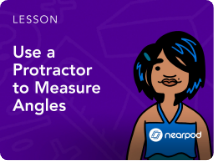#### In this collection of fourth grade resources, students build toward mastery of:

• Understanding angles as an attribute of two-dimensional figures
• Estimating angle measurements, measuring angles in whole-number degrees using a protractor, and drawing angles
• Writing equations to find unknown angles when angles are decomposed into non-overlapping parts
• Identifying right, acute, and obtuse angles
• Drawing and identifying points, lines, line segments, rays, perpendicular lines, and parallel lines
• Two-dimensional figures based on the presence or absence of parallel or perpendicular lines or angles of a specified size
• Identifying line-symmetric figures and drawing lines of symmetry
•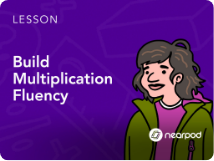#### In this collection of fifth grade resources, students build toward mastery of:

• Multiplying up to three- by two-digit whole numbers using various strategies, including the standard algorithm
• Fluently multiplying up to three- by and four- by two-digit numbers
• Using the standard algorithm to multiply up to five- by one-digit whole numbers where no or one or more new units are composed
• Dividing up to four- by two-digit whole numbers using various strategies
• Dividing up to four- by two-digit whole numbers with procedural reliability
• Representing and solving multi-step problems involving the four operations with whole numbers
•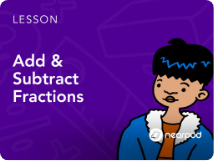#### In this collection of fifth grade resources, students build toward mastery of:

• Adding and subtracting fractions with unlike denominators using benchmark numbers and models
• Adding and subtracting fractions with unlike denominators by replacing given fractions with equivalent fractions
• Adding and subtracting fractions and mixed numbers with unlike denominators by replacing given fractions with equivalent fractions
• Adding and subtracting fractions with unlike denominators by solving real-world problems
•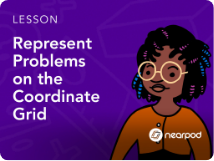#### In this collection of fifth grade resources, students build toward mastery of:

• Understanding coordinate planes by exploring the attributes of Quadrant I
• Plotting points on the coordinate plane in Quadrant I
• Interpreting and completing number patterns and sequences
• Interpreting patterns in tables using coordinate planes
• Interpreting the first quadrant of coordinate planes to solve real-world problems
• Interpreting and creating scatterplots
•#### In this collection of sixth grade resources, students build toward mastery of:

• Modeling and writing ratios involving images and diagrams
• Writing and simplifying ratios given real-world scenarios
• Converting mathematical and real-world ratios into unit rates, and using unit rates to solve real-world problems
• Identifying equivalent ratios
• Using models & double number lines to show equivalent ratios and solve real-world problems
• Solving part-part-whole problems with ratio
• Creating and using tables that represent ratios
• Completing tables with ratio relationships and graphing the corresponding ordered pairs
• Solving ratio problems that involve constant speeds and unit prices
•#### In this collection of sixth grade resources, students build toward mastery of:

• Evaluating numerical expressions involving whole-number exponents
• Evaluating algebraic expressions for specific integer values
• Writing algebraic expressions given descriptions, writing descriptions given algebraic expressions, and identifying parts of expressions using mathematical terms
• Writing equivalent expressions using the distributive property and factoring using models
• Writing equivalent expressions algebraically
• Identifying two equivalent expressions
• Determining if given integer values make a one-step equation true
• Writing one-step expressions from verbal descriptions
• Writing and solving one-step equations with and without models
•#### In this collection of sixth grade resources, students build toward mastery of:

• Composing and decomposing shapes to determine area
• Calculating the area of parallelograms and triangles
• Calculating the area of composite figures made of squares, rectangles, and triangles, and using the area of composite figures to solve real-world problems
• Calculating the volume of cubes and prisms with fractional side lengths to solve real-world and mathematical problems
• Identifying nets of three-dimensional shapes and using nets to determine surface area
• Using surface area to solve real-world problems
• Finding the surface area of a cube using the surface area formula
•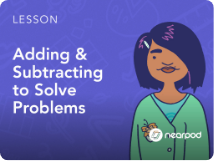#### In this collection of seventh grade resources, students build toward mastery of:

• Identifying and creating situations in which quantities combine to make zero
• Solving addition and subtraction problems, and using number lines to add rational numbers
• Fluently adding and subtracting rational numbers
• Determining when the product and quotient of two integers using properties of operations
• Fluently multiplying and dividing rational numbers
• Converting fractions to decimals using long division
• Solving both mathematical and word problems using the four operations
•#### In this collection of seventh grade resources, students build towards mastery of:

• Finding and using unit and non-unit rates to solve problems
• Identifying and writing percent change equations
• Solving percent increase and decrease problems
• Problem solving by finding original cost, tax, tip, discount cost, discount percentage, or final amount
• Solving percentage problems involving commissions, interest, and markups
• Solving measurement error, percent error, and error interval problem
•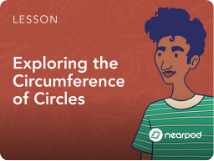#### In this collection of seventh grade resources, students build toward mastery of:

• Identifying parts of circles and finding the measure of the radius or diameter
• Calculating the circumference and area of circles and finding the radius and diameter
• Using the circumference of circles to solve word problems
• Using the area of circles to solve word problems
• Finding the area of composite figures that contain circles
• Determining area or circumference, and determining if verbal descriptions require finding area or circumference
•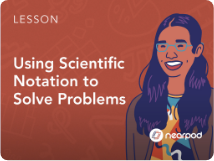#### In this collection of eighth grade resources, students build toward mastery of:

• Converting between standard numbers and scientific notation as well as comparing numbers written in scientific notation
• Adding, subtracting, multiplying, and dividing numbers written in scientific notation
• Choosing units of appropriate size for measurements of very small or very large quantities written in scientific notation
•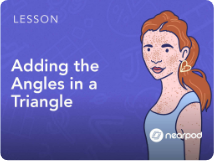#### In this collection of eighth grade resources, students build toward mastery of:

• Using the angle-sum theorem and using the exterior-angle theorem
• Calculating missing angle measurements in transversals of parallel lines
• Identifying similar triangles using the angle-angle criterion
• Understanding what the Pythagorean theorem shows
• Applying the Pythagorean theorem in mathematical and real-world problems
• Using the Pythagorean theorem to determine distances between two points on coordinate planes
•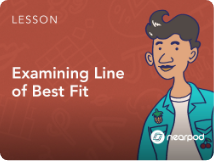#### In this collection of eighth grade resources, students build toward mastery of:

• Constructing scatter plots and describing patterns of association including clusters, outliers, positive and negative associations, and linear and nonlinear associations
• Informally fitting lines of best fit to scatter plots and assessing given lines of fit
• Using and interpreting linear equations to solve bivariate measurement problems
• Constructing two-way frequency tables, and using relative frequencies in tables to describe associations

## The supplemental math program for you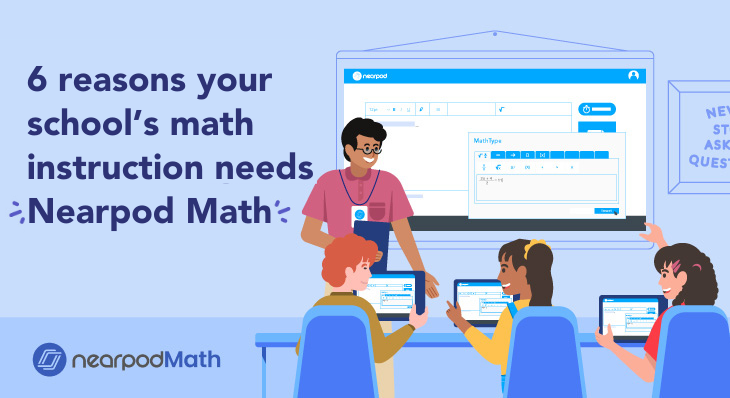Nearpod Math’s new and exciting approach to supplementing math instruction using standards-aligned math content.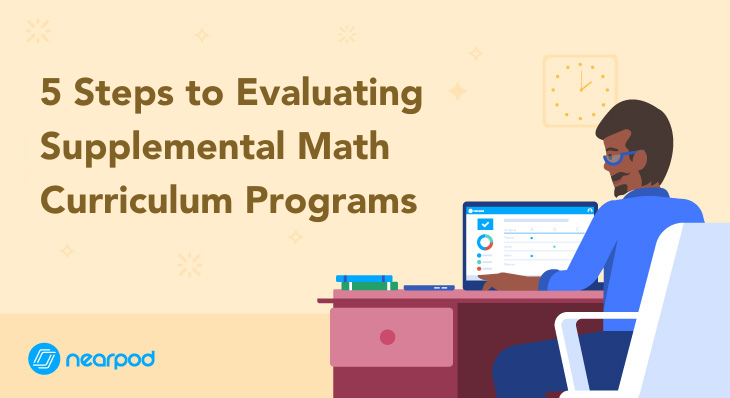Implement a suplemental curriculum program that is right for you using our 5 steps and evaluation rubric.